# Mathematics Paper 2 Questions and Answers - Form 4 End Term 1 Exams 2021

MATHEMATICS
FORM 4
END TERM EXAMS
TERM 1 2021
PAPER 2
TIME 2½ hrs

INSTRUCTIONS

• This paper has two sections: Section I and II
• Answer all questions in Section I and and FIVE in Section II

SECTION I (50MARKS)
Answer all the questions in this section in the spaces provided.

1. The length and width of a rectangular window pane measured to the nearest millimeter are 8.6cm and 5.3 respectively. Find to four significant figures, the percentage error in the area of the window pane. (3mks)(Answer to 4 significant figures)
2. Without using a calculator or mathematical tables, express in surd form and simplify leaving your answer in the form a + b √c where a, b and c are rational numbers. (3mks)
Cos 30º
Tan 45 + √3
3. In the figure below, O is the centre of the circle which passes through the points T,C and D. Line TC is parallel to OD and line ATB is a tangent to the circle at T. Angle DOC = 380. Calculate the size of angle CTB (3mks)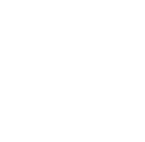4. A coffee dealer mixes two brands of coffee, x and y, to obtain 40kg of the mixture worth Ksh. 65 per kg. If brand x is valued at Ksh. 70 per kg and brand y at ks.55 per kg. Calculate the ratio, in its simplest form, in which the brands x and y are mixed. (2mks)
5. Find the radius and the coordinate of the centre of the circle whose equation is
2x2 + 2y2 – 6x + 10y + 9 = 0 (3mks)
6.
1. Expand (1 + ¼ x)4 (2mks)
2. Use your expansion in (a) above to evaluate (0.975)4 to 4 significant figures. (2mks)
7. When Ksh. 60,000 was invested in a certain bank for 8years it earned a simple interest of Ksh. 14,400. Find the amount that must have been invested in the same bank at the same rate for 5years to earn a simple interest of Ksh. 12,000 (3mks)
8. Given that P = 2q – r, express q in terms of p and r (3mks)
q + 3r
→                              →
9. If OA = 3i + 2j – 4k and OB = 4i + 5j – 2k, P divides AB in the ratio 3:-2. Determine the modulus of OP leaving your answer to 1 decimal place. Given that O is the origin. (3mks)
10. Solve for x in 2 +log7(3x-4) =log798 (3mks)
11. A carpenter wishes to make, a ladder with 18 cross-pieces. The cross pieces are to diminish uniformly in lengths from 65cm at the bottom to 31cm at the top. Calculate the length in cm, of the eighth cross-piece from the bottom. (3mks)
12. A quantity P varies partly as Q and partly as the square root of Q, given that P=30 when Q=9, and P=14 when Q=16. Find P when Q=36. (3mks)
13. Seven people can build five huts in 30 days. Find the number of people, working at the same rate that will build 9 similar huts in 27days. (3mks)
14.
1. A and B are two points on earth’s surface and on latitude 400 N. The two points are on the longitude 500W and 1300E respectively. Calculate the distance from A to B along a parallel of latitude in kilometers. (2mks)
2. The shortest distance from A to B along a great circle in kilometres (Take π = 22/7 and radius of the earth = 6370km) (2mks)
15. Find the inverse of the matrix shown hence find the coordinates of the point Of intersection of the lines
3x + y = 4 and 2x – y = 1 (3mks)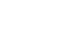16. Evaluate (4mks)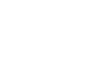SECTION II
Answer any Five questions in this section

1. The following are marks scored by form four student in Mathematics test.
 Marks 10-19 20-29 30-39 40-49 50-59 60-69 70-79 80-89 90-99 Frequency 2 6 10 16 24 20 12 8 2
Using an assumed mean of 54.5, calculate the
1. Mean mark (4mks)
2. Variance (4mks)
3. Standard deviation (2mks)
2. A bag contains 5 red, 4 white and 3 blue beads. Three beads are selected at random without replacement. Find the probability that
2. The beads selected were, white and blue: (2mks)
1. In that order
2. In any order (2mks)
3. No red bead is picked (2mks)
4. Beads picked are of the same colour. (2mks)
3. The figure below shows solid frustum of a pyramid with a square top of side 6cm and a square base of side 10cm. The slant edge of the frustum is 8cm.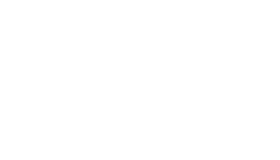1. Calculate the total surface area of the frustum (4mks)
2. Calculate the volume of the solid frustum. (3mks)
3. Calculate the angle between the planes BCHG and the base EFGH. (3mks)
4.
1. Using a ruler and pair of compasses only construct triangle ABC in which AB = 6.5cm, BC= 5.0cm and angle ABC = 600. Measure AC (3mks)
2. On same side of AB as C (3mks)
1. Determine the locus of a point P such that angle APB = 600 (3mks)
2. Construct the locus of R such that AR = 3cm. (1mk)
3. Identify the region T such that AR ≥ 3 and ∠APB ≥ 600 by shading the unwanted part. (3mks)
5. The table below shows income tax rates
 Monthly income Tax Rate (Kshs) (%) Up to 9680 10 9681 – 18800 15 18801-27920 20 27921-37040 25 37041 and above 30
Omari’s monthly taxable income is Ksh. 24200
1. Calculate the tax charged on Omari’s monthly earnings. (4mks)
2. Omari is entitled to the following tax relief of 15% of the premium paid.
Calculate the tax Omari pays each month if he pays a monthly insurance premium of Ksh. 2400 (2mks)
3. During a certain month, Omari received additional earnings which were taxed at 20% each shilling. Given that he paid 36.3% more tax that month, calculate the percentage increase in his earning. (4mks)
6. The curve of the equation y = x+ 2x2, has x = ½ and x = 0 as x-intercepts. The area bounded by the x-axis, x = ½ and x = 3 is shown by the sketch below.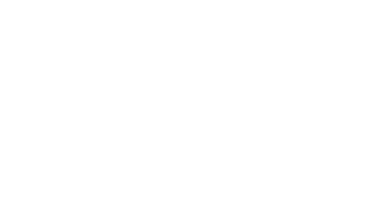Find

1.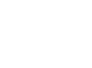2. The exact area bounded by the curve, x axis (7mks)
7.
1. Fill in the table below to 2 decimal places for the graph of y = sin x and y = 2sin (x-30) for the range
– 180 ≤ x ≤180 (2mks)
 xº -180 -150 -120 -90 -60 -30 0 30 60 90 120 150 180 Sin xº 0 -1 -0.87 0 0.87 0.5 2 Sin (x – 30)º 1 -1.73 -2 -1 1 1.73
2. On a graph, using a scale of 1cm to represent 300 on the x-axis and 1cm to represent 0.5 units on the y-axis, draw the graph of y= Sin x0 and y = 2 sin(x – 30)0 on the same axes (4mks)
1. State the amplitude and the period of the graph y = 2 sin (x-30)0 (1mk)
2. Solve the equation
Sin xº = 2 sin (x-30)º (1mk)
3. Describe fully the transformation that will map y = 2sin (x-30)0 on y = sin x (2mks)
8. A tailor makes two types of garments A and B. Garment A requires 3 metres of material while garment B requires 2 ½ metres of material. The tailor uses not more than 600 metres of material daily in making both garments. He must make not more than 100 garments of type A and not less than 80 of type B each day.
1. Write down all the inequalities from this information. (3mks)
2. Graph the inequalities in (a) above (3mks)
3. If the business makes a profit of shs. 80 on garment A and a profit of shs. 60 on garment B, how many garments of each type must it make in order to maximize the total profit? (4mks)## MARKING SCHEME

SECTION I (50MARKS)
Answer all the questions in this section in the spaces provided.

1. The length and width of a rectangular window pane measured to the nearest millimeter are 8.6cm and 5.3 respectively. Find to four significant figures, the percentage error in the area of the window pane. (3mks)(Answer to 4 significant figures)
• Maximum area ⇒ 8.65 x 5.35 = 46.2775
Actual area ⇒ 8.6 x 5.3 = 45.58
Minimum area ⇒ 8.55 x 5.25 = 44.8875
Absolute error = 46.2275 – 44.8875
2

% error = 0.695 x 100%
45.58
= 1.525%

2. Without using a calculator or mathematical tables, express in surd form and simplify leaving your answer in the form a + b √c where a, b and c are rational numbers. (3mks)
Cos 30º
Tan 45 + √3

√ 3/2
1 +√ 3

√ 3        = √ 3(2-2√ 3)
2 + 2√ 3     (2 + 2 √3 ) (2-2√3)
-2/8√3 + 3/4
= 3/41/4 √3

3. In the figure below, O is the centre of the circle which passes through the points T,C and D. Line TC is parallel to OD and line ATB is a tangent to the circle at T. Angle DOC = 380. Calculate the size of angle CTB (3mks)< OCT = 38º
< TOC = 102º
< CTB = 51º

4. A coffee dealer mixes two brands of coffee, x and y, to obtain 40kg of the mixture worth Ksh. 65 per kg. If brand x is valued at Ksh. 70 per kg and brand y at ks.55 per kg. Calculate the ratio, in its simplest form, in which the brands x and y are mixed. (2mks)
70x + 55y = 65(x +y)
5x = 10y
x : y = 2:1

5. Find the radius and the coordinate of the centre of the circle whose equation is
2x2 + 2y2 – 6x + 10y + 9 = 0 (3mks)

(x – 3/2)2 + (y +5/2)2 = 16/4
Center = (9,t) = (3/2 – 5/2) = (1.5, -2.5)

r = √ 16/4 = 2
Or
x2 + y2 – 3x + 5y + 9/2 = 0
x2 + y2 + 29x + 2fy + c = 0
-2g = -3 -2f = 5
G = 3/2 f = -5/2
Radius r =√ (f2 + g2 - c) = 25/4 + 9/49/2
r = √ 16/4 = 2

6.
1. Expand (1 + ¼ x)4 (2mks)
• (14 + 4x 1/4x) + 6 (1/4x)2 + 4(1/4x)3 + (1/4x)4
1 + 1/x + 3/8x2 + 1/6x3 + 1/6x4
2. Use your expansion in (a) above to evaluate (0.975)4 to 4 significant figures. (2mks)
• 1 + 1/-10 + 3/8(-10)2 + 1/16(-10)3 + 1/64(-10)4

• = 0.903689063
= 0.9037

7. When Ksh. 60,000 was invested in a certain bank for 8years it earned a simple interest of Ksh. 14,400. Find the amount that must have been invested in the same bank at the same rate for 5years to earn a simple interest of Ksh. 12,000 (3mks)
• 60000 x 8xt = 14400
100
T = 3 years.
P x 5 x 3 = 12000
100
P = 80,000

8. Given that P = 2q – r, express q in terms of p and r (3mks)
q + 3r
• p(q + 3r) = 2q – r
pq + 3pr = 2q – r
pq -2q = -r – 3pr
q(p-2)=-r-3pr
q=-r-3pr
p-2

→                              →
9. If OA = 3i + 2j – 4k and OB = 4i + 5j – 2k, P divides AB in the ratio 3:-2. Determine the modulus of OP leaving your answer to 1 decimal place. Given that O is the origin. (3mks)
Let p be point (x, y, z)
x               3         4
1/3   y + 2/3    2      = 5
Z             -4         -2

P(6,11,2)
10p1 = √ (6)2 + (11)2 + (2)2
= √ 161 = 12.69 units

10. Solve for x in 2 +log7(3x-4) =log798 (3mks)
• Log72 + log7 (3x-4) = log7 98
49 (x - 4) = 98
3x – 4 = 2
3x = 6
X = 2

11. A carpenter wishes to make, a ladder with 18 cross-pieces. The cross pieces are to diminish uniformly in lengths from 65cm at the bottom to 31cm at the top. Calculate the length in cm, of the eighth cross-piece from the bottom. (3mks)
31 = 65 + 17d ⇒ 17d = -34
d = -2
T8 = 65 + 7(-2)
= 65 – 14
= 51

12. A quantity P varies partly as Q and partly as the square root of Q, given that P=30 when Q=9, and P=14 when Q=16. Find P when Q=36. (3mks)
• Let L and K be constants.
P=LQ + K √Q
30 = 9L + √9K
14 = 162 + √16 K
2K + 62 = 20
2K + 8L = 7
-2L = 13
L = -13/2
K + 3(-13/2) = 10

K = 10/1 + 392 = 59/2
P = -13/2Q = 59/2 √Q

P = -13/2 x 36 + 59/2 x √36
P = -234 + 177 = -57

13. Seven people can build five huts in 30 days. Find the number of people, working at the same rate that will build 9 similar huts in 27days. (3mks)
People        Huts         days
7                5              30
9              27
7 x 30/27 x 9/5
= 14 people
14.
1. A and B are two points on earth’s surface and on latitude 400 N. The two points are on the longitude 500W and 1300E respectively. Calculate the distance from A to B along a parallel of latitude in kilometers. (2mks)
• d = 180/360 x 2 x 6370 x 22/7 Cos 40º
= 1/2 x 22/7 x 2 4879.7
= 15336.2 km

2. The shortest distance from A to B along a great circle in kilometres (Take π = 22/7 and radius of the earth = 6370km) (2mks)
• 100/360 x 2 6370 x 22/7
= 11122.2
15. Find the inverse of the matrix shown hence find the coordinates of the point Of intersection of the lines
3x + y = 4 and 2x – y = 1 (3mks)Determinant
(3 x-1)-(2x1)=(-3-2)=-5
-1/5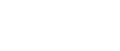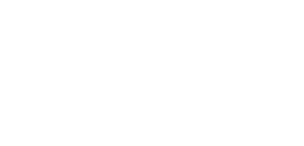16. Evaluate (4mks)(1-x)(1 +x) = 1 – x
1 + x
3
∫ (1-x)dx
-2
x – x2/2 + c
3 - 32 /2 + c – 2 – (-2)2 + c
= 3 – 4.5 + c - -2 + 2 + c
-1.5 + c – c
= -1.5

SECTION II
Answer any Five questions in this section

1. The following are marks scored by form four student in Mathematics test.
 Marks 10-19 20-29 30-39 40-49 50-59 60-69 70-79 80-89 90-99 Frequency 2 6 10 16 24 20 12 8 2
Using an assumed mean of 54.5, calculate the
1. Mean mark (4mks)
 Marks Mp(x) d(x-a) Fd D2 Fd2 10-19 2 14.5 -40 -80 1600 3200 20-29 6 24.5 -30 -180 900 5400 30-39 10 34.5 -20 -200 400 4000 40-49 16 44.5 -10 -160 100 1600 50-59 24 54.5 0 0 0 0 60-69 20 64.5 10 200 100 200 70-79 12 74.5 20 240 400 4800 80-89 8 84.5 30 240 900 7200 90-99 2 94.5 40 80 1600 3200 140 31400

Mean = A + Σfd
Σf
= 54.5 + 140/100 = 55.9

2. Variance (4mks)
• Variance= 31400/100 – (140/100)2
= 312.04

3. Standard deviation (2mks)
• standard varaitaion = √variance
√ 312.04 = 17.06
2. A bag contains 5 red, 4 white and 3 blue beads. Three beads are selected at random without replacement. Find the probability that
• P(WWR or WBR or BBR or BWR)
(4/12 X 3/11 X 5/10) + 4/12 X 3/11 X 5/10
+ (3/12 x 2/11 x 5/10 + (3/12 x 4/11 x 5/10))
= 2/11

2. The beads selected were, white and blue: (2mks)
1. In that order
5/12 x 4/11 x 3/10 = 1/22

2. In any order (2mks)
• P(RWB or RBW or WBR or WRB or BWR or BRW)
(5/12 x 4/11 x 3/10) + (5/12 x 3/11 X 4/10)
+ (4/12 x 3/11 x 5/10)
= 6/52 = 3/11

3. No red bead is picked (2mks)
• P(BBB or BBW or BWB or BWW or WWW or WWB or WBW OR WBB)
(3/12 x 2/11 x 1/10)+(3/12x2/11x4/10)+(3/12x4/11x2/10)+(3/12x4/11x3/10 )+
(4/12x3/11x2/10)+(4/12x3/11x3/10) +(4/12x3/11x2/10)
=7/44

4. Beads picked are of the same colour. (2mks)
• P(BBB or WWW or RRR)
(3/12x2/11x1/10)+(4/12x3/11x2/10)+(5/12 x 4/11x3/10)
= 1/220 + 1/55 + 1/55 = 9/220

3. The figure below shows solid frustum of a pyramid with a square top of side 6cm and a square base of side 10cm. The slant edge of the frustum is 8cm.1. Calculate the total surface area of the frustum (4mks)
• 6/10 =l/8 +L
12 = L
Base area = 102 = 100
Area of 4Δs = 4√25(25-20)(25-20)(25-20)
= 4√25 x 5 x 5x 15
= 4√ 9375
T.S.A of the pyramid = 100 + 387.28
= 487.28cm2
Area of the slanting edges of thr small pyramid
= 4√15(3)(3)(9)
= 139.44
Surface of the solid frustrum
= 487 .28 +36-139.44
383.84

2. Calculate the volume of the solid frustum. (3mks)
• Volume = 1/3 x 100 x 18.71
= 62.61
L.S.F = 3/5 ⇒ V.S.F 27/125
Fraction representing Frustrum
= 98/125
∴Volume of the frustrum = 98/125 x 623.61
= 488.91
3. Calculate the angle between the planes BCHG and the base EFGH. (3mks)
• tanα=18.71
5
α = 75.03º
4.
1. Using a ruler and pair of compasses only construct triangle ABC in which AB = 6.5cm, BC= 5.0cm and angle ABC = 600. Measure AC (3mks)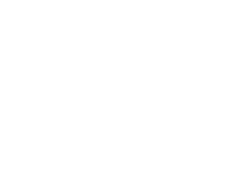AC=5.8±1
2. On same side of AB as C (3mks)
1. Determine the locus of a point P such that angle APB = 60º (3mks)
2. Construct the locus of R such that AR = 3cm. (1mk)
3. Identify the region T such that AR ≥ 3 and ∠APB ≥ 60º by shading the unwanted part. (3mks)
5. The table below shows income tax rates
 Monthly income Tax Rate (Kshs) (%) Up to 9680 10 9681 – 18800 15 18801-27920 20 27921-37040 25 37041 and above 30
Omari’s monthly taxable income is Ksh. 24200
1. Calculate the tax charged on Omari’s monthly earnings. (4mks)
Tax on 1st ksh 9680 = 9680 x 10/100 = kshs. 968
Tax on 2nd kshs. 9120 = 9120 x 15/100= kshs 1368
Tax on rem kshs. 5400 = 5400 x 20/100= ksh 1080
Total tax = 968 + 1368 + 1080 = kshs. 3416

2. Omari is entitled to the following tax relief of 15% of the premium paid.
Calculate the tax Omari pays each month if he pays a monthly insurance premium of Ksh. 2400 (2mks)
• Tax paid= 3416-(1056 + 2400 x 15/100)
= kshs. 2000

3. During a certain month, Omari received additional earnings which were taxed at 20% each shilling. Given that he paid 36.3% more tax that month, calculate the percentage increase in his earning. (4mks)
• Increase in tax paid = 2000 x 36.3= kshs. 726
Increase in earnings= kshs. 726 x 100/20= ksh. 3630
% increase = 3630 x 100
24200

6. The curve of the equation y = x+ 2x2, has x = ½ and x = 0 as x-intercepts. The area bounded by the x-axis, x = ½ and x = 3 is shown by the sketch below.Find

1.x2/2 + 2/3x3 + c

2. The exact area bounded by the curve, x axis (7mks)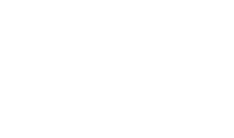=0-(1/81/12)
= - (3-2)
24
= - 1/241/24
32/2 + 2/3 x 33 __(0)

9/2 + 54/3
4.5 + 18 = 22.5
Total area = 1/24 + 22.5=0.0416 + 22.5
= 22.54
7.
1. Fill in the table below to 2 decimal places for the graph of y = sin x and y = 2sin (x-30) for the range
– 180 ≤ x ≤180 (2mks)
 xº -180 -150 -120 -90 -60 -30 0 30 60 90 120 150 180 Sin xº 0 -0.5 -0.87 -1 -0.87 -0.5 0 0.5 0.87 1 0.87 0.5 0 2 Sin (x – 30)º 1 0 -1 -1.73 -2 0 -1 0 1 1.73 2 1.73 1
2. On a graph, using a scale of 1cm to represent 300 on the x-axis and 1cm to represent 0.5 units on the y-axis, draw the graph of y= Sin x0 and y = 2 sin(x – 30)0 on the same axes (4mks)

1. State the amplitude and the period of the graph y = 2 sin (x-30)0 (1mk)
• y = 2sin(x- 30º)
Amplitude = 3units
Period = 360º

2. Solve the equation
Sin xº = 2 sin (x-30)º (1mk)
SinX = 2Sin (x – 30º)

X = -126º cr 51.50 ±1º

3. Describe fully the transformation that will map y = 2sin (x-30)0 on y = sin x (2mks)
+30
0 Translation

8. A tailor makes two types of garments A and B. Garment A requires 3 metres of material while garment B requires 2 ½ metres of material. The tailor uses not more than 600 metres of material daily in making both garments. He must make not more than 100 garments of type A and not less than 80 of type B each day.
1. Write down all the inequalities from this information. (3mks)
(i) 3x + 2 1/2y ≤ 600
(ii) x ≤ 100
(iii) Y ≥ 80, x ≥ 0

2. Graph the inequalities in (a) above (3mks)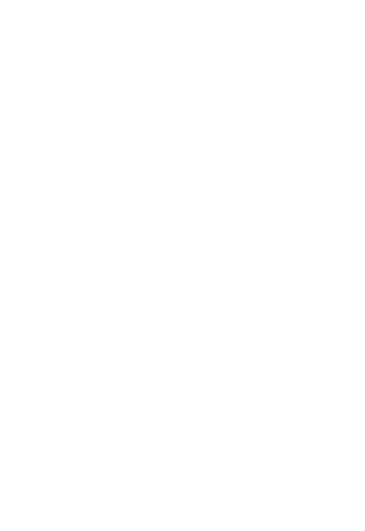line 3x + 2 1/2y ≤ 600
Line x ≤ 100
Line ≥ 80, x ≥ 0

3. If the business makes a profit of shs. 80 on garment A and a profit of shs. 60 on garment B, how many garments of each type must it make in order to maximize the total profit? (4mks)
The objective functions
P = 80x + 60y
100 garments of type A
120 garments of type B

• ✔ To read offline at any time.
• ✔ To Print at your convenience
• ✔ Share Easily with Friends / Students